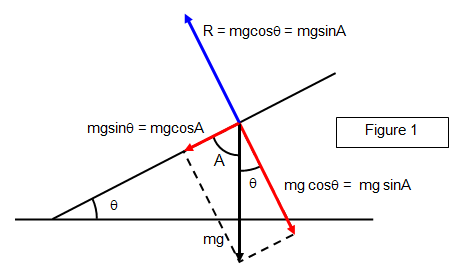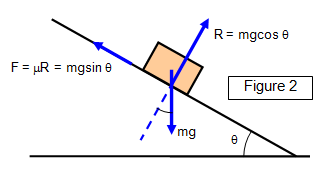# The inclined planeThe components of the forces acting on a point object (P) on an inclined plane are shown in Figure 1. The actual forces are the weight of the object represented by the black vector and the reaction of the plane, shown by the blue vector. The components of the weight are shown in red.

An inclined plane may be used to measure the coefficient of friction between two surfaces.

The object is placed on a slope as shown in Figure 2 and the tilt of the slope (θ) is slowly increased until the object begins to slide down.At the moment of slip the forces on the object are given by:

along the plane: F = mg sin q
perpendicular to the plane: R = mg cos q

Therefore:

Coefficient of friction (m) = F/R = tanq

A VERSION IN WORD IS AVAILABLE ON THE SCHOOLPHYSICS USB